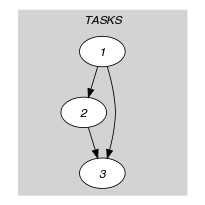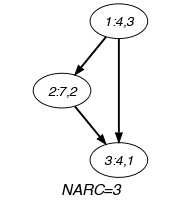## 5.128. disjunctive_or_same_start

Origin

Scheduling.

Constraint

$\mathrm{𝚍𝚒𝚜𝚓𝚞𝚗𝚌𝚝𝚒𝚟𝚎}_\mathrm{𝚘𝚛}_\mathrm{𝚜𝚊𝚖𝚎}_\mathrm{𝚜𝚝𝚊𝚛𝚝}\left(\mathrm{𝚃𝙰𝚂𝙺𝚂}\right)$

Synonyms

$\mathrm{𝚜𝚊𝚖𝚎}_\mathrm{𝚜𝚝𝚊𝚛𝚝}_\mathrm{𝚘𝚛}_\mathrm{𝚍𝚒𝚜𝚓𝚞𝚗𝚌𝚝𝚒𝚟𝚎}$, $\mathrm{𝚗𝚘𝚗}_\mathrm{𝚘𝚟𝚎𝚛𝚕𝚊𝚙}_\mathrm{𝚘𝚛}_\mathrm{𝚜𝚊𝚖𝚎}_\mathrm{𝚜𝚝𝚊𝚛𝚝}$, $\mathrm{𝚜𝚊𝚖𝚎}_\mathrm{𝚜𝚝𝚊𝚛𝚝}_\mathrm{𝚘𝚛}_\mathrm{𝚗𝚘𝚗}_\mathrm{𝚘𝚟𝚎𝚛𝚕𝚊𝚙}$.

Argument
 $\mathrm{𝚃𝙰𝚂𝙺𝚂}$ $\mathrm{𝚌𝚘𝚕𝚕𝚎𝚌𝚝𝚒𝚘𝚗}\left(\mathrm{𝚘𝚛𝚒𝚐𝚒𝚗}-\mathrm{𝚍𝚟𝚊𝚛},\mathrm{𝚍𝚞𝚛𝚊𝚝𝚒𝚘𝚗}-\mathrm{𝚍𝚟𝚊𝚛}\right)$
Restrictions
 $\mathrm{𝚛𝚎𝚚𝚞𝚒𝚛𝚎𝚍}$$\left(\mathrm{𝚃𝙰𝚂𝙺𝚂},\left[\mathrm{𝚘𝚛𝚒𝚐𝚒𝚗},\mathrm{𝚍𝚞𝚛𝚊𝚝𝚒𝚘𝚗}\right]\right)$ $\mathrm{𝚃𝙰𝚂𝙺𝚂}.\mathrm{𝚍𝚞𝚛𝚊𝚝𝚒𝚘𝚗}\ge 0$
Purpose

All pairs of tasks of the collection $\mathrm{𝚃𝙰𝚂𝙺𝚂}$ that have a duration strictly greater than 0 should either not overlap either have the same start, i.e. $\forall i\in \left[1,|\mathrm{𝚃𝙰𝚂𝙺𝚂}|\right],\forall j\in \left[i+1,|\mathrm{𝚃𝙰𝚂𝙺𝚂}|\right]:$ $\mathrm{𝚃𝙰𝚂𝙺𝚂}\left[i\right].\mathrm{𝚍𝚞𝚛𝚊𝚝𝚒𝚘𝚗}=0\vee \mathrm{𝚃𝙰𝚂𝙺𝚂}\left[j\right].\mathrm{𝚍𝚞𝚛𝚊𝚝𝚒𝚘𝚗}=0\vee \mathrm{𝚃𝙰𝚂𝙺𝚂}\left[i\right].\mathrm{𝚘𝚛𝚒𝚐𝚒𝚗}+\mathrm{𝚃𝙰𝚂𝙺𝚂}\left[i\right].\mathrm{𝚍𝚞𝚛𝚊𝚝𝚒𝚘𝚗}\le \mathrm{𝚃𝙰𝚂𝙺𝚂}\left[j\right].\mathrm{𝚘𝚛𝚒𝚐𝚒𝚗}\vee \mathrm{𝚃𝙰𝚂𝙺𝚂}\left[j\right].\mathrm{𝚘𝚛𝚒𝚐𝚒𝚗}+\mathrm{𝚃𝙰𝚂𝙺𝚂}\left[j\right].\mathrm{𝚍𝚞𝚛𝚊𝚝𝚒𝚘𝚗}\le \mathrm{𝚃𝙰𝚂𝙺𝚂}\left[i\right].\mathrm{𝚘𝚛𝚒𝚐𝚒𝚗}\vee \mathrm{𝚃𝙰𝚂𝙺𝚂}\left[i\right].\mathrm{𝚘𝚛𝚒𝚐𝚒𝚗}=\mathrm{𝚃𝙰𝚂𝙺𝚂}\left[j\right].\mathrm{𝚘𝚛𝚒𝚐𝚒𝚗}$.

Example
$\left(\begin{array}{c}〈\begin{array}{cc}\mathrm{𝚘𝚛𝚒𝚐𝚒𝚗}-4\hfill & \mathrm{𝚍𝚞𝚛𝚊𝚝𝚒𝚘𝚗}-3,\hfill \\ \mathrm{𝚘𝚛𝚒𝚐𝚒𝚗}-7\hfill & \mathrm{𝚍𝚞𝚛𝚊𝚝𝚒𝚘𝚗}-2,\hfill \\ \mathrm{𝚘𝚛𝚒𝚐𝚒𝚗}-4\hfill & \mathrm{𝚍𝚞𝚛𝚊𝚝𝚒𝚘𝚗}-1\hfill \end{array}〉\hfill \end{array}\right)$

Since the starts of the first and third tasks coincide, and since the second task does neither overlap the first task nor the third task, the $\mathrm{𝚍𝚒𝚜𝚓𝚞𝚗𝚌𝚝𝚒𝚟𝚎}_\mathrm{𝚘𝚛}_\mathrm{𝚜𝚊𝚖𝚎}_\mathrm{𝚜𝚝𝚊𝚛𝚝}$ constraint holds.

Typical
 $|\mathrm{𝚃𝙰𝚂𝙺𝚂}|>2$ $\mathrm{𝚃𝙰𝚂𝙺𝚂}.\mathrm{𝚍𝚞𝚛𝚊𝚝𝚒𝚘𝚗}\ge 1$
Symmetries
• Items of $\mathrm{𝚃𝙰𝚂𝙺𝚂}$ are permutable.

• $\mathrm{𝚃𝙰𝚂𝙺𝚂}.\mathrm{𝚍𝚞𝚛𝚊𝚝𝚒𝚘𝚗}$ can be decreased to any value $\ge 0$.

• One and the same constant can be added to the $\mathrm{𝚘𝚛𝚒𝚐𝚒𝚗}$ attribute of all items of $\mathrm{𝚃𝙰𝚂𝙺𝚂}$.

Arg. properties

Contractible wrt. $\mathrm{𝚃𝙰𝚂𝙺𝚂}$.

Keywords
Arc input(s)

$\mathrm{𝚃𝙰𝚂𝙺𝚂}$

Arc generator
$\mathrm{𝐶𝐿𝐼𝑄𝑈𝐸}$$\left(<\right)↦\mathrm{𝚌𝚘𝚕𝚕𝚎𝚌𝚝𝚒𝚘𝚗}\left(\mathrm{𝚝𝚊𝚜𝚔𝚜}\mathtt{1},\mathrm{𝚝𝚊𝚜𝚔𝚜}\mathtt{2}\right)$

Arc arity
Arc constraint(s)
$\bigvee \left(\begin{array}{c}\mathrm{𝚝𝚊𝚜𝚔𝚜}\mathtt{1}.\mathrm{𝚍𝚞𝚛𝚊𝚝𝚒𝚘𝚗}=0,\hfill \\ \mathrm{𝚝𝚊𝚜𝚔𝚜}\mathtt{2}.\mathrm{𝚍𝚞𝚛𝚊𝚝𝚒𝚘𝚗}=0,\hfill \\ \mathrm{𝚝𝚊𝚜𝚔𝚜}\mathtt{1}.\mathrm{𝚘𝚛𝚒𝚐𝚒𝚗}+\mathrm{𝚝𝚊𝚜𝚔𝚜}\mathtt{1}.\mathrm{𝚍𝚞𝚛𝚊𝚝𝚒𝚘𝚗}\le \mathrm{𝚝𝚊𝚜𝚔𝚜}\mathtt{2}.\mathrm{𝚘𝚛𝚒𝚐𝚒𝚗},\hfill \\ \mathrm{𝚝𝚊𝚜𝚔𝚜}\mathtt{2}.\mathrm{𝚘𝚛𝚒𝚐𝚒𝚗}+\mathrm{𝚝𝚊𝚜𝚔𝚜}\mathtt{2}.\mathrm{𝚍𝚞𝚛𝚊𝚝𝚒𝚘𝚗}\le \mathrm{𝚝𝚊𝚜𝚔𝚜}\mathtt{1}.\mathrm{𝚘𝚛𝚒𝚐𝚒𝚗},\hfill \\ \mathrm{𝚝𝚊𝚜𝚔𝚜}\mathtt{1}.\mathrm{𝚘𝚛𝚒𝚐𝚒𝚗}=\mathrm{𝚝𝚊𝚜𝚔𝚜}\mathtt{2}.\mathrm{𝚘𝚛𝚒𝚐𝚒𝚗}\hfill \end{array}\right)$
Graph property(ies)
$\mathrm{𝐍𝐀𝐑𝐂}$$=|\mathrm{𝚃𝙰𝚂𝙺𝚂}|*\left(|\mathrm{𝚃𝙰𝚂𝙺𝚂}|-1\right)/2$

Graph model

We generate a clique with a non-overlapping constraint or a same start constraint between each pair of distinct tasks and state that the number of arcs of the final graph should be equal to the number of arcs of the initial graph.

Parts (A) and (B) of Figure 5.128.1 respectively show the initial and final graph associated with the Example slot. The $\mathrm{𝚍𝚒𝚜𝚓𝚞𝚗𝚌𝚝𝚒𝚟𝚎}_\mathrm{𝚘𝚛}_\mathrm{𝚜𝚊𝚖𝚎}_\mathrm{𝚜𝚝𝚊𝚛𝚝}$ constraint holds since all the arcs of the initial graph belong to the final graph.

##### Figure 5.128.1. Initial and final graph of the $\mathrm{𝚍𝚒𝚜𝚓𝚞𝚗𝚌𝚝𝚒𝚟𝚎}_\mathrm{𝚘𝚛}_\mathrm{𝚜𝚊𝚖𝚎}_\mathrm{𝚜𝚝𝚊𝚛𝚝}$ constraint(a) (b)